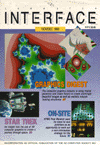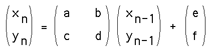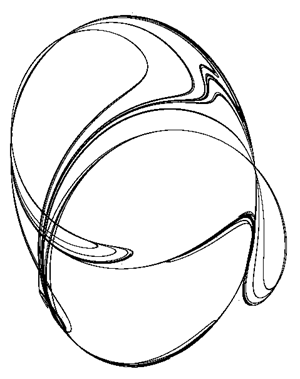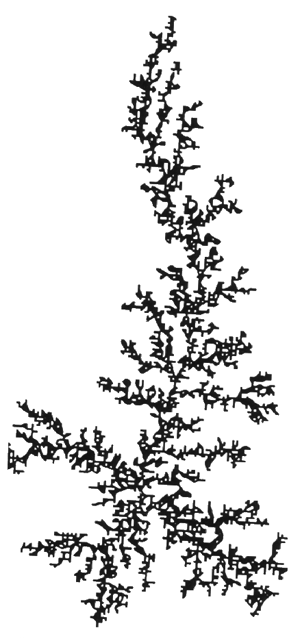# Fractals and Computer Graphics

Appeared in Interface Magazine, December 1990

Written by Paul Bourke### Introduction

Computer graphics using CAD software is typically good at creating representations of man-made objects using primitives such as lines, rectangles, polygons, and curves in 2 D or boxes and surfaces in 3D. These geometric primitives and usual tools for manipulating them typically prove inadequate when it comes to representing most objects found in nature such as clouds, trees, veins, waves, and a clump of mud. There has been considerable interest recently in chaos theory and fractal geometry as we find that many processes in the world can be accurately described using that theory. The computer graphics industry is rapidly incorporating these techniques to generate stunningly beautiful images as well as realistic natural looking structures.

In what follows a description of a few of the more commonly used techniques will be given along with an example of each. It should be appreciated that usually the example is one from an image with a large if not infinite variation depending on the parameters, scale, and viewing position.

### Chaotic Systems

The classic Mandelbrot, figure 1, has been the image that has greatly popularised chaotic and fractal systems. The Mandelbrot set is created by a general technique where a function of the form

zn+1 = f(zn)

is used to create a series of a complex variable. In the case of the Mandelbrot, the function is

f(zn) = zn2 + zo

This series is generated for every initial point zo on some partition of the complex plane. To draw an image on a computer screen the point under consideration is coloured depending on the behaviour of the series which will act in one of the following ways:

(a) decay to 0
(b) tend to infinity
(c) oscillate among a number of states
(d) exhibit no discernible pattern

In figure 1, situation (a) occurs in the interior portion, (b) in the exterior, (c) and (d) near the boundary. The boundary of the set exhibits infinite detail and variation (the boundary will never appear smooth irrespective of the zoom factors). as well as self-similarity.

An example using the same technique but a different function is called biomorphs by CA Pickover. It uses the function

f(zn) = sin(zn2) + c

and gives rise to many biological looking creatures depending on the value of the constant c, see figure 2. Another example resulting in galactic swirls is obtained using the function

f(zn) = u zn(1 - zn).

Figure 3 shows part of the function with

u = -0.7 + 0.8i

### Strange Attractors

A second technique called hopalong is normally used to represent the strange attractor of a chaotic system, for example, the well known Julia set. In this case each coordinate generated by the series is drawn as a small point, ie: we hop-along from one point to the next. For an image on a plane the series is either an equation of a complex variable or else there are two interrelated equations, one of the x and one for y coordinate. As an example consider the following function:

xn+1 = yn - sign(xn)|b xn - c|0.5

yn+1 = a - xn

This series of the x,y coordinates is specified by an initial point x0,y0 and three constants a, b, and c. As an example see figure 4 where a=0.4, b=1, and c=0. Interestingly, for strange attractors the initial point does not matter (except for a few special cases), ie: all initial coordinates x0,y0 result in the same image. In other words, the image shows the x,y pairs that can be generated by the series, any initial point will generate the same set of points although they will generated in a different order.

Another example attributed to Peter de Jong uses the two equations:

xn+1 = sin(a yn) - cos(b xn)
yn+1 = sin(c xn) - cos(d yn)

This gives swirling tendrils that appear three dimensional, an example is shown in figure 5 where a = -2.23, b = -0.65, c = 0.43, d = -2.43.

### Newton Raphson

This technique is based on the Newton Raphson method of finding the solution (roots) to a polynomial equation of the form

f(z) = ao + a1 z + a2z2 + ... + am zm = 0

The method generates a series where the n+1'th approximation to the solution is given by

zn+1 = zn - f(zn) / f'(zn)

where f'(zn) is the slope (first derivative) of f(z) evaluated at zn.

To create a 2D image using this technique each point in a partition of the plane is used as initial guess, z0, to the solution. The point is coloured depending on which solution is found and/or how long it took to arrive at the solution. A simple example is an application of the above to find the three roots of the polynomial z3 - 1 = 0.

Figure 6 shows the appearance of a small portion of the positive real and imaginary quadrant of the complex plane. A trademark of chaotic systems is that very similar initial conditions can give rise to very different behaviour. In the image shown there are points very close together, one of which converges to the solution very fast and the other converges very slowly.

### Diffusion Limited Aggregation

Many attractive images can be generated using theory from areas of chemistry and physics. One such example is diffusion limited aggregation or DLA which describes, among other things, the diffusion and aggregation of zinc ions in an electrolytic solution onto electrodes. Another more colourful description involves a city square surrounded by taverns. Drunks leave the taverns and stagger randomly around the square until they finally trip over one their insensate companions at which time lulled by the sounds of peaceful snoring they lie down and fall asleep. The tendril like structure is an aerial view of the sleeping crowd in the morning, see figure 7.

### L-Systems

Very recently there has been renewed interest in Lindemayer or simply L-Systems. This is a set of string rewriting rules which takes an initial string of characters called the axiom and on every iteration replaces each of the characters in the string by other strings called production rules. For example consider the axiom string: F+F+F+F and the single production rule F-->F+F-F-FF+F+F-F

Now if some characters are giving geometric meaning then the string can be drawn. Using these geometric meanings for the symbols an example of an axiom, production rule, and the resulting string after a few iterations, the result is the well known Koch curve which has a fractal dimension between 1 and 2. Production rules can specify the full range of classical fractal curves (for example the van Koch snowflake, space filling curves such as the Hilbert and Peano curves, the dragon curve, as well as kolam patterns).

Recent usage of L-Systems is for the creation of realistic looking objects that occur in nature and in particular the branching structure of plants. Three examples of plant structures generated from L-Systems are illustrated in figure 8.

One of the important characteristics of L-Systems is that only a small amount of information is required to represent very complex objects. So while the bushed in figure 8 contains many thousands of lines, they can be described in a database by only a few bytes of data, the actual bushes are only grown when required for visual presentation. Using suitably designed L-System algorithms it is possible to design the L-System production rule that will create a particular class of plant.

### Iterated Function Systems

Instead of working with lines as in L systems, IFS replaces polygons by other polygons as described by a generator. On every iteration each polygon is replaced by a suitably scaled, rotated, and translated version of the polygons in the generator.

From this geometric description it is also possible to derive a hopalong description which gives the image that would be created after iterating the geometric model to infinity. The description of this is a set of contractive transformations on a plane to the form below each with an assigned probability.To run the system an initial point is chosen and on each iteration one of the transformation is chosen randomly accordingly to the assigned probabilities, the resulting points (xn,yn) are drawn on the page.

As in the case of L systems, if the IFS code for a desired image can be determined (by something called Collage theorem) then large data compression ratios can be achieved. Instead of storing the geometry of the very complex object, just the IFS generator needs to be stored and the image can be generated when required.

### Summary

The real application of much of the above has arisen from attempts to model natural phenomena in the world we live in. Many of the mathematical techniques have found a firm place in the computer graphics industry as a means of creating both stunning graphical images as well as very natural looking structures. As the techniques become more standardised and more application areas are found they are likely to be incorporated as one of the standard tools in CAD, painting and image processing software packages.

For further reading on this topic a highly recommended book is the Science of Fractal Images by H.O. Peitgen and D. Saupe, published by Springer-Verlag, 1988. All of these systems as well as many others are available form the author for the Macintosh family of computers.Figure 1

```

```Figure 2

```

```Figure 3

```

```Figure 4: Barry Martin, Scientific American 1986

```

```Figure 5

```

```Figure 6

```

```Figure 7

```

```Figure 8